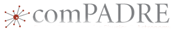|

# Measuring the coefficient of friction of a skater on iceThis material was originally developed through comPADRE
as part of its collaboration with the SERC Pedagogic Service.

#### Summary

Students use a video clip of a gliding ice skater to determine the coefficient of friction of the skater on ice. Vernier LoggerPro's video analysis tools are used to plot the velocity vs time. From the slope of this line, students can determine the acceleration and use Newton's Second Law to calculate the coefficient of sliding friction between the skates and the ice.

## Learning Goals

A video clip of kids gliding across ice contains all the information needed to determine to coefficient of friction between skates and ice.
Students learn that they can extract sufficient data from a simple video clip of a skater gliding across ice to determine the coefficient of sliding friction. This type of activity is a departure from conventional textbook questions where all the required quantities are provided explicitly.

Students also practice video analysis skills, graphing, data interpretation, and Newtonian mechanics.

## Context for Use

This activity is appropriate for use in an introductory physics course, either high school or college level. It is best used with students who have some experience using Vernier's LoggerPro video analysis tools. The student handout provides step-by-step instructions, but the exercise could also be presented as an open-ended activity.

## Teaching Materials

Outline of activity:
2. Students import the video into LoggerPro, use the video analysis tools to capture frame-by-frame data of the skater's motion.
3. Next, students plot the horizontal velocity vs time for the skater during the period that the skater is gliding. The slope of this graph shows the acceleration -- the rate at which the skater is slowing due to the force for friction of the ice on the skates.
4. Then, students use Newton's second Law along with the definition of the coefficient of friction to develop a relationship between the acceleration of an object being slowed by friction and the coefficient of friction.
5. Finally student use this relationship and the acceleration from their graph to calculate the coefficient of friction between the skates and the ice.

Materials:

• A word document that describes the process. instructions for the ice skate friction acivity (Microsoft Word 44kB Aug19 10) Note that this document provides specific step-by-step instructions. You may want to present this as more of an open-ended problem.
• Six video clips of children gliding on skates. The video clips include a reference object that can be used to scale the video. The video clips show students gliding in both directions across the ice so students can compare the values for right-going skaters to left-going skaters to determine whether factors such as an incline or wind influenced the results.
1. Mari glides to the right ice skating video 1 (Quicktime Video 883kB Aug19 10)
2. Mari glides to the left ice skating video 2 (Quicktime Video 870kB Aug19 10)
3. Siri glides to the right ice skating video 3 (Quicktime Video 811kB Aug19 10)
4. Siri glides to the left ice skating video 4 (Quicktime Video 865kB Aug19 10)
5. Carl glides to the right ice skating video 5 (Quicktime Video 772kB Aug19 10)
6. Carl glides to the left ice skating video 6 (Quicktime Video 763kB Aug19 10)
• A sample LoggerPro file showing the video analysis process. LoggerPro file of ice skate friction ( 34kB Aug19 10)

• A sample graph of the velocity vs time for a skater gliding, showing the slope (acceleration). The graph shows velocity vs time for a skater gliding across the ice. The slope of this graph is the acceleration -- rate at which the skater slows due to the force of friction. This acceleration can be used to calculate the coefficient of friction between the skates and ice.

## Teaching Notes and Tips

• Students need to have access to Vernier's LoggerPro data analysis software. This software is available from Vernier.com and cost \$189 for a school site license (which includes students' personal computers) at the time of this writing.
• Ideally, this activity would be conducted in an environment in which each student has access to their own computer. Students download one of the video files provided and do their own analysis. Students would present and compare their results.
• Note that this could be taught as more of an open-ended exercise than is shown in the word document. For example, students could be shown one of the video clips and challenged to develop a method to determine the coefficient of friction between skates and ice.
• See a complete description of how to teach with video clips, including pedagogic strategies and a collection of sample videos.

## Assessment

Possible assessments include:
• students write a traditional lab report
• students produce a short video lab report describing the process and their results
• students complete a set of questions (see student worksheet provided in teaching materials)

## References and Resources

Vernier , manufacturer of LoggerPro data analysis software
Instructions on doing video analysis using LoggerPro (pdf file)
A description of a similar problem. In the problem shown, we find the maximum acceleration for a car on a surface with a given coefficient of friction. In the activity described here, we use the acceleration to calculate the coefficient of friction.